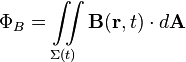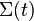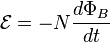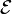# Faraday's law of induction facts for kids

Kids Encyclopedia Facts

Faraday's law of induction is a law of physics proposed by English physicist Michael Faraday in 1831. It is one of the basic laws of electromagnetism. The law explains why generators, transformers and electrical motors work.

Faraday's law of induction says that when a magnetic field changes, it causes a voltage, a difference in the electric potential that can make electric currents flow. That phenomenon was also found by Joseph Henry in 1831.

Imagine we have a closed loop of wire. To figure out how much current will be "induced" (i.e. produced by the magnetic field), we need to define the magnetic flux, a number describing how much of the magnetic field is actually going through the loop. Magnetic fields are vector fields, so they have both a strength and a direction. This leads to the following surface integral:$\Phi_B = \iint\limits_{\Sigma(t)} \mathbf{B}(\mathbf{r}, t) \cdot d \mathbf{A}$

where

• ΦB is the magnetic flux
•$\Sigma(t)$is the (possibly moving) surface whose boundary is the wire loop
• B is the magnetic field
• dA is a small part of the surface.

That is, we could imagine filling in the wire loop with a thin surface, like a soap film. This formula tells us to look at every point on that surface, measure how much the magnetic field is pointing straight through the loop at that point, and add up all those measurements to get a single number. That number is the magnetic flux. When the flux changes, it produces electromotive force. The flux changes when B changes or when the wire loop is moved or bent, or when both happen. The electromotive force can then be calculated with the following equation:$\mathcal{E} = -N {{d\Phi_B} \over dt}$
•$\mathcal{E}$ is the electromotive force
• N is the number of loops the wire makes
• ΦB is the magnetic flux of one loop
• - is representative of Lenz's law and indicates direction of the electromotive force

This equation says that how much current is induced in the wire loop depends directly on how fast the magnetic flux is changing in time, whether due to the loop moving or the magnetic field changing.

## Images for kids

de:Elektromagnetische Induktion#Induktionsgesetz in IntegralformFaraday's law of induction Facts for Kids. Kiddle Encyclopedia.